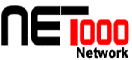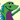You are here:  Home > Science > Math > Statistics
• Allstat Statistics Mailing List - Allstat is a UK-based worldwide e-mail broadcast system for the statistical community, operated by CTI Statistics and maintained by Dr Stuart G Young.
• Bureau of Labor Statistics
• Chance Database Welcome Page - This data base contains materials designed help teach a Chance course or a more standard introductory probability or statistics course.
• History of Monte Carlo method - The Monte Carlo method provides approximate solutions to a variety of mathematical problems by performing statistical sampling experiments on a computer.
• HyperStat - an introductory-level hypertext statistics book.
• Interactive Statistical Calculations Page - This is an organized collection of over 300 links to web pages that perform statistical calculations. The collection is organized into sections like a statistical software package, covering probability functions, descriptive statistics, group comparisons (including ANOVA), regression & correlation, cross-tabs, power & sample size calculations, etc. It is, in effect, a free, multi-platform, universally-accessible statistical software package. The website also includes extensive links to downloadable software, online textbooks and tutorials, and other statistical resources.
• InterStat - Open source of articles about statistics.
• Modern Regression and Classification - 2 day course for overview of computer-based regression and classification.
• Mx - a matrix algebra interpreter and numerical optimizer for structural equation modeling and other types of statistical modeling of data
• National Science Foundation - Statistics and Probability Program
• The pLab Project Home Page - A server on the theory and practice of random number generation.
• Power Analysis for ANOVA Designs
• Power Calculator - returns distribution values based on user inputs for many common statistical distributions.
• The Probability Web - a collection of pages to serve people with interests in probability theory and its applications.
• Random Number Generation, Taygeta Scientific Inc. - information and articles on random number generation.
• Statistical analysis - Principles of mixed model analysis. Includes discussion of REML estimation of variances, BLUEs and BLUPs, fixed and random effects, and inference space; with small examples.
• Statistical Inference on the TI-82/83 - guides to statistical features of the TI-82/83 graphing calculators.
• Statistical List Subscription Service - An easy-to-use, form-based way of subscribing to and unsubscribing from a variety of statistical e-mail discussion lists.
• Statistics Every Writer Should Know - This easy-to-understand guide offers short lessons (with examples!) in basic mathematics for journalists and other writers.
• The Statistics Homepage - This Electronic Statistical Textbook offers training in the understanding and application of statistics.
• Statistics to Use - online statistics demosHelp build the largest human-edited directory of the Web - Become an Editor